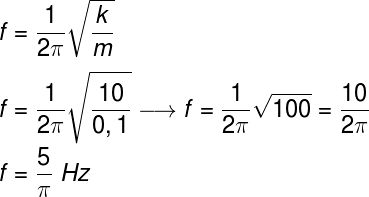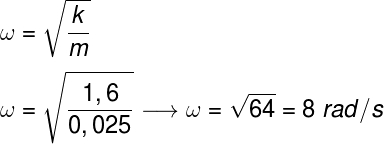Mechanics

# spring-mass oscillator

Spring-mass oscillator is a physical system composed of an ideal spring, with spring constant k, attached to a body of mass m. When stretched or compressed, the spring acquires elastic potential energy , when released, this potential energy is periodically converted into kinetic energy .

## spring-mass system

The spring-mass system is one of the simplest types of harmonic oscillators . When the spring has its original length changed, a restoring force of elastic origin acts on it, so that it returns to its equilibrium position.

### Abstract: important concepts about the spring-mass system

Elongation (x): is the measure of the change in the length of the spring, based on its initial length. In this sense, the elongation can either be positive, when the spring is stretched, or negative, when the spring is compressed;

Amplitude (A): is the greatest possible elongation for the spring-mass oscillator. Amplitude, like elongation, also admits of negative values;

Period (T): is the time required for a spring-mass system to complete one oscillation. This time is proportional to the spring constant of the spring (k), which is measured in newtons per meter (N/m), according to SI ;

Frequency (f): is the number of oscillations that the mass-spring system completes every second, it is a quantity of dimension s -1 (second -1 ), measured in hertz (Hz);

Angular velocity (ω): measures how quickly the phase of the oscillatory motion varies, it is also known as angular frequency or pulsation, its unit is the radian per second (rad/s);

Initial phase (θ 0 ): it is the measure of the phase in which the harmonic oscillator movement is in the initial instant of time.

## Vertical spring-mass system

It is common to see exercises in which the mass-spring system is vertical, and it is possible to solve them in the same way as if it were arranged in the horizontal direction, since the same equations are valid for both cases.

## Spring-mass oscillator equations

In addition to the basic equations used to write any and all simple harmonic motion (SHM), the mass-spring system has some special mathematical relationships related to the spring constant of the spring and the mass of the body in which the spring is attached.

The mass-spring oscillator equations can be obtained based on Newton’s 2nd law and Hooke’s law. To do so, it is necessary to realize that the resultant force on the body of mass m is an elastic force, therefore, this force is equivalent to the product of the mass of the body and the acceleration :

For this calculation, it was necessary to take into account that the acceleration a can be calculated as ω²x. This is one of the relationships from the general time equations of the MHS.

Based on the result obtained, it is possible to write the other equations for the mass-spring oscillator , check:

The above equations can be used to calculate the mass-spring oscillator frequency and period , respectively.

## Energy in the spring-mass oscillator

In the simple spring-mass oscillator there is no frictional force or any other forces capable of dissipating energy. In this way, the total mechanical energy of the system remains constant throughout the movement. This mechanical energy, in turn, is calculated by adding the kinetic energy to the elastic potential energy.

Conservation of mechanical energy in the mass-spring system

## Mass-spring oscillator exercises

Question 1) A spring with a spring constant of 10 N/m is attached to a mass of 100 g (0.1 kg). When compressed, this spring starts to oscillate, describing a simple harmonic motion. Determine the frequency of oscillation of the set.

a) 5 Hz

b) 5π Hz

c) 5/π Hz

d) 4π Hz

e) 3/π Hz

Template : letter c

Resolution :

To solve the exercise, we will use the frequency formula for the mass-spring oscillator, check:According to the calculation, the mass-spring oscillation frequency is 5/π Hz.

Question 2) A spring with a spring constant of 1.6 N/m is attached to a mass of 0.025 kg. After a stimulus, the set starts to oscillate in simple harmonic motion. Determine the angular frequency of the motion.

Template: letter c

Resolution:

This exercise requires us to apply the formula for the angular velocity of the mass-spring oscillator, note:The calculation indicates that the angular velocity of the movement is 8 radians per second, so the correct alternative is the letter c.

Question 3) Regarding the motion described by an ideal spring-mass system, free from any dissipative forces, mark the correct alternative:

a) The kinetic energy of this motion remains constant.

b) The frequency of the movement is proportional to the mass of the body that is attached to the spring.

c) The period of this motion depends directly on the acceleration of local gravity.

d) In this type of system, the mechanical energy does not change, since there is no presence of dissipative forces.

e) The elastic potential energy in this type of movement only decreases.

Template: letter d

Resolution:

Let’s analyze the alternatives:

a) FALSE – Since the velocity changes along the oscillatory motion, the kinetic energy related to that motion also changes.

b) FALSE – The frequency is inversely proportional to the square root of the body’s mass.

c) FALSE – The movement whose period depends on local gravity is the simple pendulum.

d) TRUE

e) FALSE – As with velocity, elastic potential energy both increases and decreases.